# Bases for Integration from First Principles

## Introduction to Integration from First Principles

Foreword: This paper introduces you to my discovery of Integration from First Principles. (Refresh your page in case all the formulas are not downloaded)

By: Chrysanthus Date Published: 21 Mar 2019

### Introduction

Integration is area under the curve.

Consider the following diagram: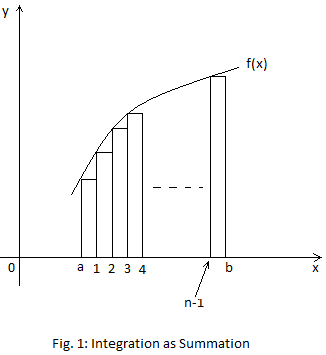y = f(x)

You might have seen the following: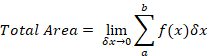This statement is not perfect. It should actually be: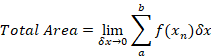where f(xn) is a particular height from the curve to the x-axis.

My Discovery

The widths of the vertical strips are equal.

As 𝛿x turns to 0, the number of strips increases.

n is the total number of strips.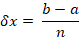As 𝛿x → 0, n → ∞.

The series and the formula for its summation, for all the heights of the strips from a to b, is obtained. If you do not know the formula for the series, develop one, and that may take time. Summing can be done for the heights, from 0 to n-1.

The summation expression above in the statement, is replaced with the summation formula.

As n → ∞, the right-hand-side of the statement becomes the definite integral; and that concludes the scheme.

Let total area be represented by A. So, my statement for the Integration from First Principles is: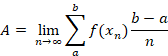Integration of the Constant, C

Let the definite integral be represented by A, and let the indefinite integral be represented by I. Let the summation expression (discrete summation) be Sn.

Here,

y = C

i.e.

f(x) = C

Sn = (C0 + C + C + C + - - - + Cn-1)𝛿x

= (nC) 𝛿x

But⸫ Sn =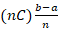The ns cancel:

=> Sn = C(b - a)

=> Cb - Ca

As n → ∞,

A = Cb -Ca ………………….the definite integral

If a is 0 and b is x,

I = Cx ………………………..the indefinite integral

Integration of y = x

That is,

f(x) = x

Sn = [a + (a+𝛿x) + (a+2𝛿x) + (a+3𝛿x) + - - - + (a+(n-1)𝛿x)]𝛿x

The series in square brackets is an Arithmetic Progression, with first term = a and common difference = 𝛿x.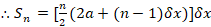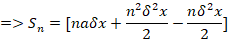But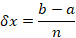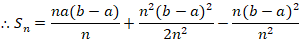As n → ∞,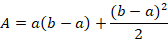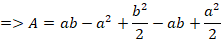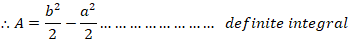If a is 0 and b is x,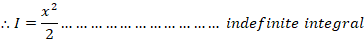Integration of y=x2

That is,

f(x) = x2

Sn = [(a+0𝛿x)2 + (a+1𝛿x)2 + (a+2𝛿x)2 + (a+3𝛿x)2 + - - - + (a+(n-1)𝛿x)2]𝛿x

=> Sn = [a2

𝛿x + 𝛿2x

+ a2 + 4a𝛿x + 4𝛿2x

+ a2 + 6a𝛿x + 9𝛿2x

+

.

.

+ a2 + 2a(n-1)𝛿x + (n-1)2𝛿2x]𝛿x

=> Sn = [a2 + a2 + a2 + a2 + - - - +aan

𝛿x + 4a𝛿x + 6a𝛿𝛿x

+ 1𝛿2x + 4𝛿2x + 9𝛿2x + - - - + (n-1)2𝛿2x]𝛿x

The first row in square brackets gives, na2.

The second row is an Arithmetic Progression, with first term = 0 and common difference = 2a𝛿x, giving,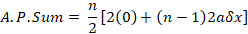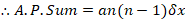The third row is,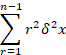A common formula for the r2 series is,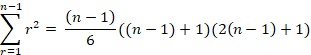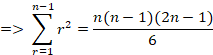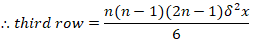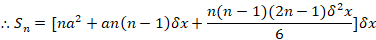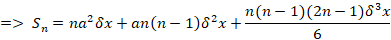But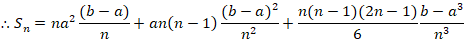As n → ∞,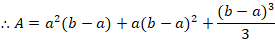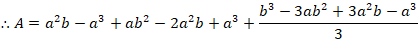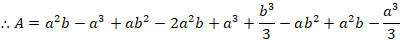After subtractions, we have,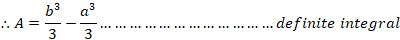If a is 0 and b is x,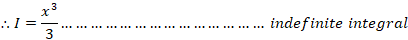Integration of y=x3

That is,

f(x) = x3

Sn = [(a+0𝛿x)3 + (a+1𝛿x)3 + (a+2𝛿x)3 + (a+3𝛿x)3 + - - - + (a+(n-1)𝛿x)3]𝛿x

=> Sn = [a3

+ a3 + 3a2𝛿x + 3a𝛿2x + 𝛿3x

+ a3 + 3(21)a2𝛿x + 3(22)a𝛿2x + (23)𝛿3x

+ a3 + 3(31)a2𝛿x + 3(32)a𝛿2x + (33)𝛿3x

+

.

.

+ a3 + 3(n-1)a2𝛿x + 3(n-1)2a𝛿2x + (n-1)3𝛿3x]𝛿x

=> Sn = [a3 + a3 + a3 + a3 + - - - +a3n

+ 3a2𝛿x + 3(31)a2𝛿x + 3(31)a2𝛿x + - - - + 3(n-1)a2𝛿x

+ 3a𝛿2x + 3(22)a𝛿2x + 3(32)a𝛿2x + - - - + 3(n-1)2a𝛿2x

+ 𝛿3x + (23)𝛿3x + (33)𝛿3x + - - - + (n-1)3𝛿3x]𝛿x

The first row in square brackets gives, na3.

The second row is an Arithmetic Progression, with first term = 0 and common difference = 3a2𝛿x, giving,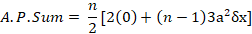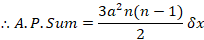The third row is,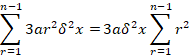A common formula for the r2 series is,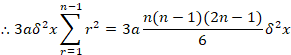The fourth row is,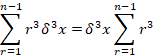A common formula for the r3 series is,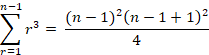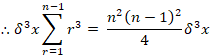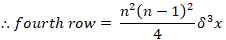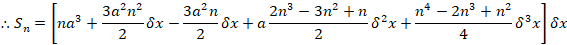ButAs n → ∞,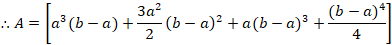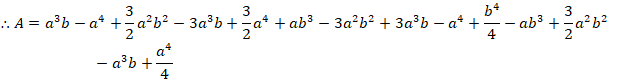After subtractions and additions, we have,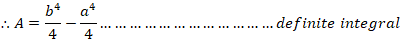If a is 0 and b is x,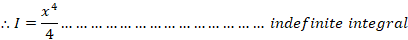Do we have to continue looking for the summation of each power series: x4, x5, x6, etc.? – No! Wait for the complete paper before the end of next month, which has my power series for xn.

The Complete Paper

I will publish the complete paper, which solves different types of integrations, taught for pure and further math in Anglo-Saxon high schools, from First Principles; before the end of next month. I will publish the full paper in this website.

Chrys